• Subject:

Physics

• Topic:

Units and Measurement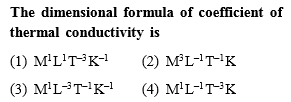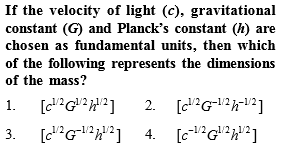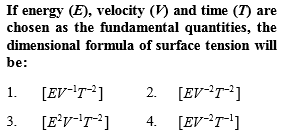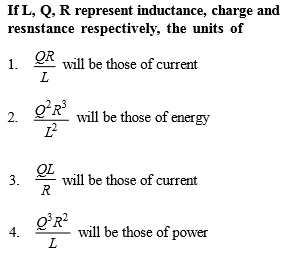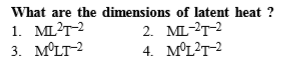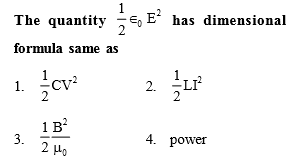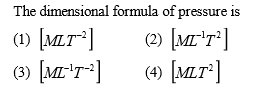Light year is a unit of

(1) Time

(2) Mass

(3) Distance

(4) Energy

PMT

The magnitude of any physical quantity

(1) Depends on the method of measurement

(2) Does not depend on the method of measurement

(3) Is more in SI system than in CGS system

(4) Directly proportional to the fundamental units of mass, length and time

Which of the following is not equal to watt

(1) Joule/second

(2) Ampere $×$ volt

(3) (Ampere)2 $×$ ohm

(4) Ampere/volt

PMT